Next: Fine Structure Up: Time-Independent Perturbation Theory Previous: Degenerate Perturbation Theory

# Linear Stark Effect

Let us examine the effect of a static, external, electric field on the excited energy levels of a hydrogen atom. For instance, consider thestates. There is a singlestate, usually referred to as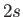, and threestates (with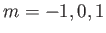), usually referred to as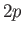. (In this notation, the 2 refers to the value of the principal quantum number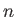, whereas,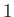,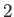,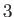,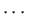are represented by the letters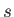,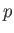,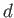,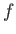,.) All of these states possess the same energy,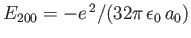. (See Section 4.6.) As in Section 7.4, the perturbing Hamiltonian is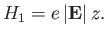(7.82)

In order to apply perturbation theory, we have to solve the matrix eigenvalue equation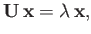(7.83)

where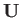is the array of the matrix elements of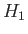between the degenerateandstates. Thus,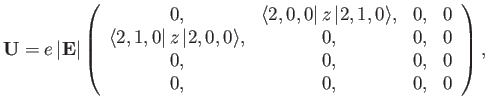(7.84)

where the rows and columns correspond to the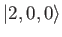,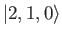,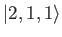, and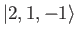states, respectively. Here, we have made use of the selection rules, which tell us that the matrix element ofbetween two energy eigenstates of the hydrogen atom is zero unless the states possess the same magnetic quantum number,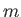, and azimuthal quantum numbers,, that differ by unity. (See Section 7.4.) It is easily demonstrated, from the exact forms of theandwavefunctions, that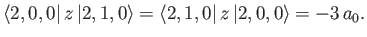(7.85)

(See Exercise 15.)

It can be seen, by inspection, that the eigenvalues ofare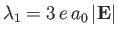,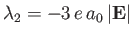,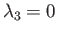, and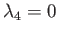. The corresponding eigenvectors are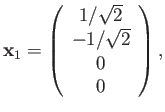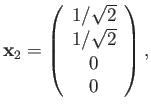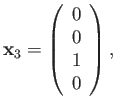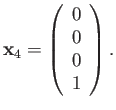(7.86)

It follows from Section 7.5 that the simultaneous eigenstates of the unperturbed Hamiltonian and the perturbing Hamiltonian take the form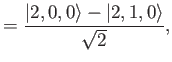(7.87)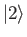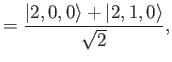(7.88)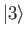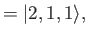(7.89)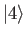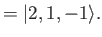(7.90)

In the absence of an external electric field, all of these states possess the same energy,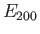. The first-order energy-shifts induced by such a field are given by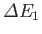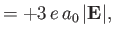(7.91)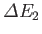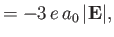(7.92)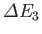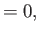(7.93)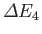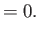(7.94)

Thus, the energies of states 1 and 2 are shifted upwards and downwards, respectively, by an amount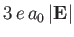, in the presence of an external electric field. States 1 and 2 are orthogonal linear combinations of the originaland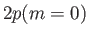states. Note that the energy-shifts are linear in the electric field-strength. Consequently, this phenomenon is known as the linear Stark effect. Of course, for weak perturbing electric fields, the linear Stark effect is much larger effect that the quadratic effect described in Section 7.4. The energies of states 3 and 4 [which are equivalent to the original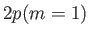and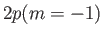states, respectively] are not affected to first order in the perturbation expansion. Of course, to second order, the energies of these states are shifted by an amount that depends on the square of the electric field-strength.

The linear Stark effect depends crucially on the degeneracy of theandstates. This degeneracy is a special property of a pure Coulomb potential, and, therefore, only applies to a hydrogen atom. (See Section 4.6.) Thus, alkali metal atoms do not exhibit the linear Stark effect.Next: Fine Structure Up: Time-Independent Perturbation Theory Previous: Degenerate Perturbation Theory
Richard Fitzpatrick 2016-01-22